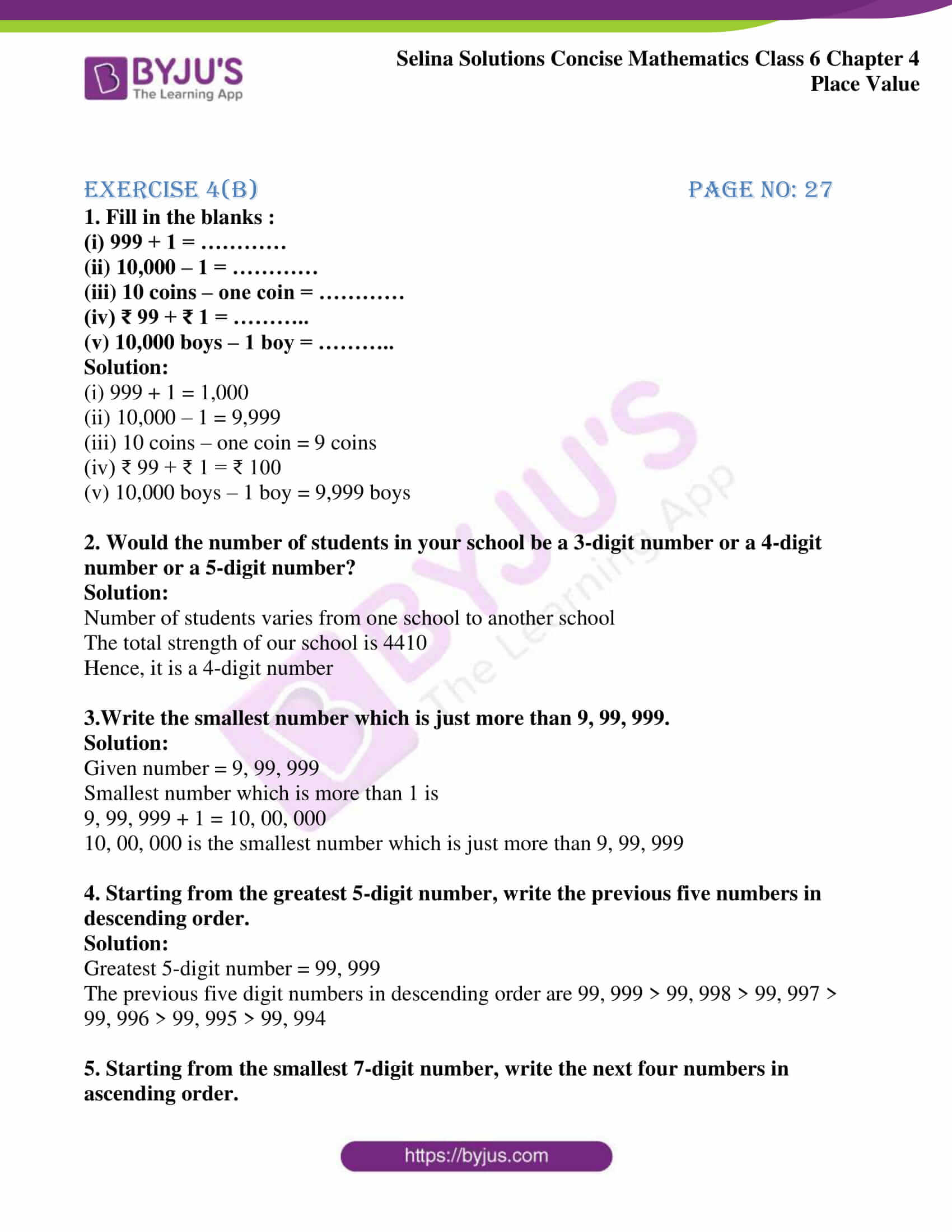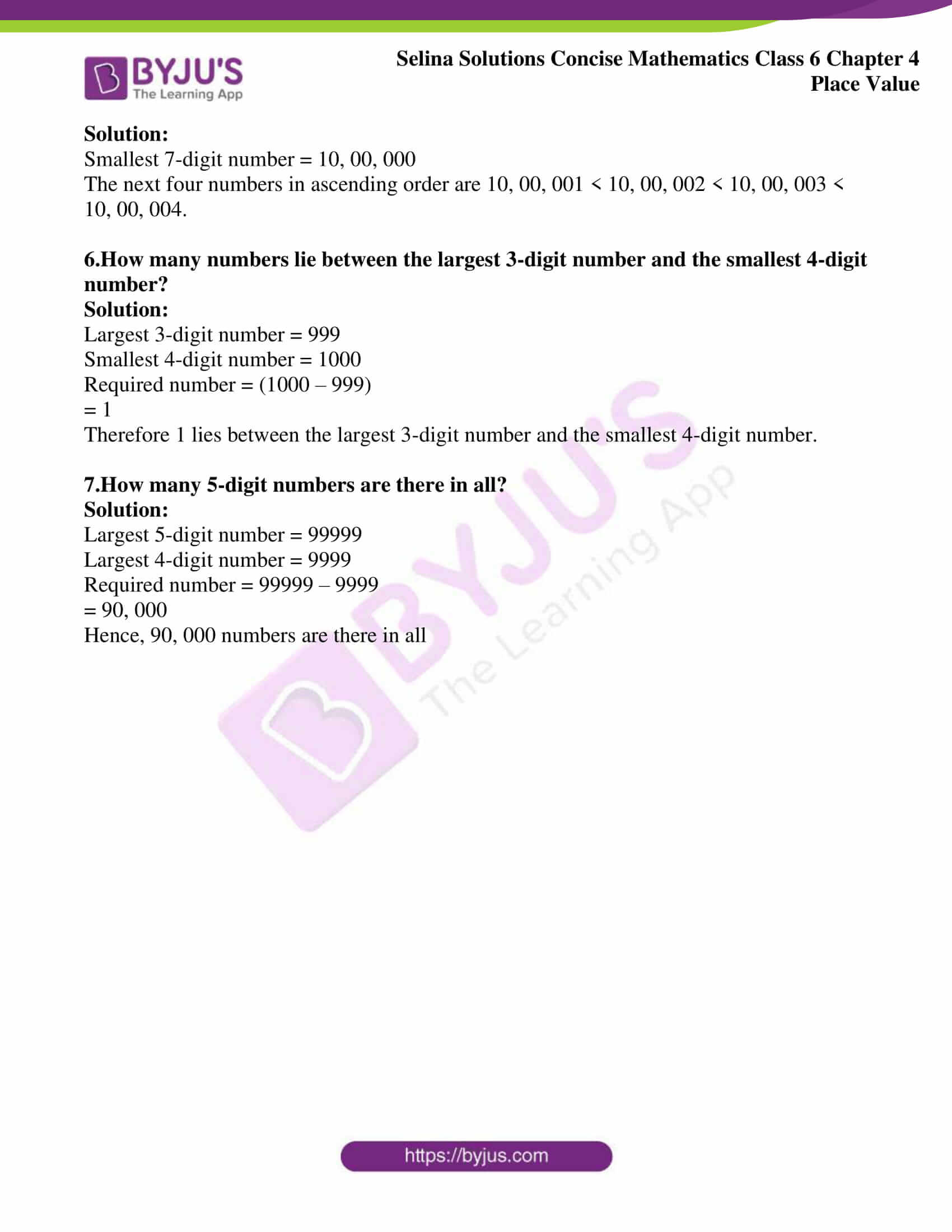# Selina Solutions Concise Mathematics Class 6 Chapter 4: Place Value Exercise 4(B)

This exercise consists of the problems based on the largest and the smallest numbers along with solved examples, as per the Selina textbook. By referring to Selina Solutions Concise Mathematics, students solve the complex problems with ease in a short duration. The Solutions PDF are helpful in improving exam preparation among students. Practising the Solutions, which are well structured by experts will boost students’ understanding capacity. Students can use Selina Solutions Concise Mathematics Class 6 Chapter 4 Place Value Exercise 4(B) PDF to download, from the links available here.

## Selina Solutions Concise Mathematics Class 6 Chapter 4 Place Value Exercise 4(B) Download PDF### Access Selina Solutions Concise Mathematics Class 6 Chapter 4: Place Value Exercise 4(B)

#### Exercise 4(b) page no: 27

1. Fill in the blanks :
(i) 999 + 1 = …………
(ii) 10,000 – 1 = …………
(iii) 10 coins – one coin = …………
(iv) ₹ 99 + ₹ 1 = ………..
(v) 10,000 boys – 1 boy = ………..

Solution:

(i) 999 + 1 = 1,000

(ii) 10,000 – 1 = 9,999

(iii) 10 coins – one coin = 9 coins

(iv) ₹ 99 + ₹ 1 = ₹ 100

(v) 10,000 boys – 1 boy = 9,999 boys

2. Would the number of students in your school be a 3-digit number or a 4-digit number or a 5-digit number?

Solution:

Number of students varies from one school to another school

The total strength of our school is 4410

Hence, it is a 4-digit number

3.Write the smallest number which is just more than 9, 99, 999.

Solution:

Given number = 9, 99, 999

Smallest number which is more than 1 is

9, 99, 999 + 1 = 10, 00, 000

10, 00, 000 is the smallest number which is just more than 9, 99, 999

4. Starting from the greatest 5-digit number, write the previous five numbers in descending order.

Solution:

Greatest 5-digit number = 99, 999

The previous five digit numbers in descending order are 99, 999 > 99, 998 > 99, 997 > 99, 996 > 99, 995 > 99, 994

5. Starting from the smallest 7-digit number, write the next four numbers in ascending order.

Solution:

Smallest 7-digit number = 10, 00, 000

The next four numbers in ascending order are 10, 00, 001 < 10, 00, 002 < 10, 00, 003 < 10, 00, 004.

6.How many numbers lie between the largest 3-digit number and the smallest 4-digit number?

Solution:

Largest 3-digit number = 999

Smallest 4-digit number = 1000

Required number = (1000 – 999)

= 1

Therefore 1 lies between the largest 3-digit number and the smallest 4-digit number.

7.How many 5-digit numbers are there in all?

Solution:

Largest 5-digit number = 99999

Largest 4-digit number = 9999

Required number = 99999 – 9999

= 90, 000

Hence, 90, 000 numbers are there in all